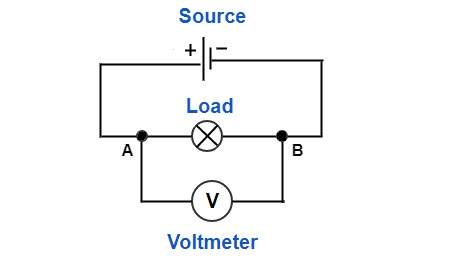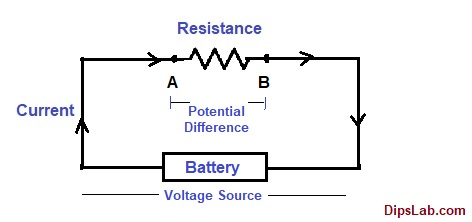# The Beginner’s Guide to Voltage | Potential Difference | EMFWhat do you think about the voltage, potential difference, and EMF?

Actually, many of us are confused about these three terms. Sometimes we think that these are the same. If these three terms are the same, why there are three different names?

These are very basic terms in Electrical and Electronics Engineering. If you understand it properly, it will help you throughout engineering.

In this post, I am explaining voltage, potential difference and electromotive force (EMF). Let’s see if they are the same or different.

### What is Voltage?

In the practical study, voltage is called as pressure (P) or tension (T).

#### Definition

Here is my simplified basic definition of the voltage so that everyone can understand it.

When a potencial is provided through the electrical power source, it pushes electrons easily.  This potencial is called as the Voltage.

The technical definition of the voltage is –

Voltage is the potential energy requires to flow the electric charge (or electrons) in an electrical circuit.

If we talk about electrical equipment, the potential energy is provided in the form of the cell or battery.

The cell or battery is an electronic device that stores chemical energy and transfers into electrical energy.

What is the difference between Cell and Battery?

The cell is a single DC (Direct Current) power generating unit. And battery consists of two or more electrochemical cells for generating more DC power.

You can see the block diagram of the energy conversion system below.Block Diagram for the Battery Conversion

The symbolic representation is different for cell and battery.Call Symbolic Representation with VoltageBattery Symbolic Representation with Voltage

#### Symbolic Representation of Voltage for DC and AC

Voltage is represented by the capital letter V.

The symbolic representation of the direct current and alternating current sources are shown in the below diagram.

• For DC SourceDirect Current (DC) Voltage Source Symbol

• For AC SourceAlternating Current (AC) Voltage Source Symbol

#### Voltage Formula

We can calculate the value of the voltage or potential difference by using the basic formula.

`Voltage =  (Electric Potential Energy / Flowing of Electric Charged Particles)  J/C`

It means that the voltage measured is the electrical potential energy per unit charge.

Where,

• Electric Potential energy (W) is expressed in Joule (J).
• Electric charge particle (Q) are expressed in Coulomb (C).

You can also put it in another format. If you are knowing current and resistance, you can calculate the voltage.

`Voltage = Current * Resistance or V = (I * R)      Volt`

Where,

• Current (I) is expressed in Ampere.
• Resistance (R) is expressed in ohm.

#### Measurement of the Voltage

The voltage is expressed in Volt.  There are electrical types of equipment to measure the voltage called as Voltmeter or Multimeter.

Why it is called as Volt?

If you look at history, it’s a bit interesting.

In 1745 – 1827, Italian physicist Alessandro Volta has invented the first electrical Voltaic pile battery.  The unit of the voltage-Volt is derived from his name.

To calculate the voltage in any electrical circuit, you have to connect a voltmeter in a parallel position.  (You can read the difference between series and parallel circuit.)

You can see the following figure where voltmeter is connected across the terminal of the load.A voltmeter connected in parallel across the load

A voltmeter is just one equipment. There are many Electrical Measuring Instruments you should be knowing.

#### Calculating Voltage using Ohm’s and Kirchhoff’s Laws

Here are two basic formulas you can use to calculate the voltage in electrical circuits.

• Ohm’s Law

According to ohm’s law, voltage is the product of the electric current and resistance.  You can calculate the value of the voltage by putting current and resistance values.

`Voltage = Current * Resistance or V = (I * R) Volt`
• Kirchhoff’s Law

According to Kirchhoff’s Voltage Law (KVL), the different voltage or equivalent voltage can be determined in a circuit.

` Ʃ (V) = (V1 + V2 + V3 + V4 +........ + Vn)`

#### Online Tool for Calculating Voltage

You can be calculated the value of voltage by using simple online tools.

Now you don’t have to bother about going wrong while doing the mathematical calculations. This is a simple explanation. I tried to sum up all the basics of the electrical voltage.

Let’s move to our other term- Potential Difference.

### What is the Difference between Voltage and Potential Difference?

Voltage and potential difference both are similar for their use of flowing charged particles. But, both works in different aspects.

Potential difference is shortly denoted by the P.D.

Simple definition.

Potential difference defines as the potential energy requires to move or transfer the unit of the charge from one place to another in between two terminal of the electrical field.

According to the definition, it is represented as

`Potencial Difference =( Energy or Work / Charge)     J/C`

The S.I. unit of the potential difference and the voltage are the same. Both are measured in voltmeter.

Lets we will study,

How does the potential difference work in the circuit?

In the given diagram, the electric circuit consists of the voltage source (battery) and resistive load. You can connect different types of an ideal and practical voltage source.The positive terminal of the battery has a higher potential and negative terminal of battery has lower potential. Both ends of the terminal are connected with the resistive load to complete the closed-loop circuit.

When the electric current passes through the resistive load, the voltage changes between two terminal.  This changing voltage is called as Voltage Drop.

The energy is required to flow of electric current from higher potential (point A) into lower potential (point B). In between two given points, the occurring voltage is called a potential difference.

### What is the Difference Between Voltage and EMF (Electromotive Force)?

EMF is nothing but the force. It is another form of energy.

It is shortly abbreviated as the EMF or emf or E.

The electromotive force is defined as the force requires to move charge particles and generate electrical energy from the other power genertaing source.

The EMF is denoted by (cursive letter E) ε. The S.I unit of the EMF is Volt.

Function:

The main function of the EMF is to,

• maintain the potential difference
• produce electrical energy from the non-electric source

Multiple electrical devices are used to generate EMF such as electrochemical cells, solar (PV) cells, electrical motor and generators, transformers, etc.

This is an explanation about the voltage, potential difference, and electromotive force. Hopefully, you understood it very well now.

If you have any queries, you can freely comment below.

### 21 thoughts on “The Beginner’s Guide to Voltage | Potential Difference | EMF”

1.Hi, I just wanted to say, I loved this blog post.
It was inspiring. Keep on posting!

•Thank you, Mick:)

2.Great methodology

3.It’s actually a helpful piece of information. I am glad that you just shared this useful information with us.
Please, keep us informed like this. Thanks for sharing.

•And I am promising that, I will share the more useful and updated post on DipsLab.

4.Good Explanation, Mam.

•Thanks, Shubham 🙂

5.Very well explanation, Mam.

•Thanks, Jagdip 🙂

6.Thank you, for your own hard work on this blog. I take pleasure in setting aside time for investing in your blog and it is simple to grasp.

You’re the one performing a wonderful job.

•Thanks, William for reaching out to my blog. And I feel very glad to hear this sound.

7.Thanks for one’s marvelous posting!

•You’re welcome, Sejal 🙂

8.I really like your writing style, good information, thank you for putting up :D.

•Thanks, Sairina 🙂

9.Thank you, Mam.

•You’re welcome, Dear 🙂

10.Hi Dipali, I visit your website on regular basis to get updated on the most recent information. Great job!

•Thanks, Parber to reach out to my blog and find out daily update.

11.•